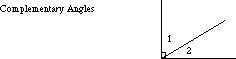index: click on a letter A B C D E F G H I J K L M N O P Q R S T U V W X Y Z A to Z index index: subject areas numbers & symbols sets, logic, proofs geometry algebra trigonometry advanced algebra & pre-calculus calculus advanced topics probability & statistics real world applications multimedia entrieswww.mathwords.com about mathwords website feedback

 Complementary Angles Two acute angles that add up to 90°. For example, 40° and 50° are complementary. In the diagram below, angles 1 and 2 are complementary.See also